Transformations of coordinates

Translation of axes. Turning around origin of coordinates.

Central symmetry. Homothetic transformation. Affine transformation.

Consider some transformations tied with a transition from one coordinate system to another. Here ( õ , ó ) è ( õ ' , ó ' ) are coordinates of arbitrary point P in old and new coordinate systems correspondingly.

Translation of axes. Let's move the coordinate system XOY in a plane so, that the axes OX and OY are parallel to themselves, and the origin of coordinates Î moves to the point Î ' ( a , b ). We'll receive the new coordinate system X'O'Y' ( Fig.1 ):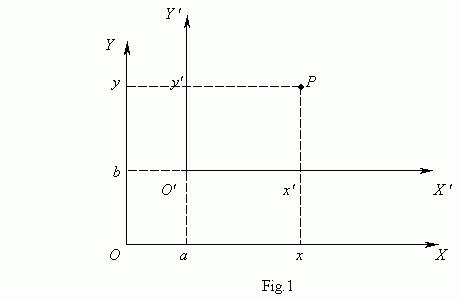Coordinates of the point P in the new and old coordinate systems are tied by the equations: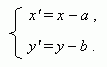Turning around origin of coordinates. Let's turn the coordinate system X Î Y in a plane by an angle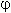( Fig.2 ).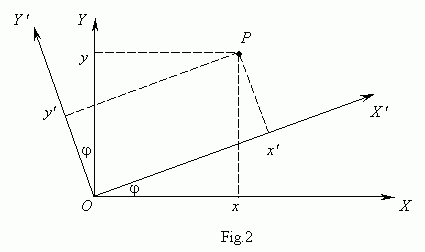Now coordinates of the point P in the new and old coordinate systems are tied by the equations: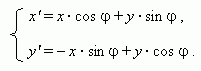In the particular case:=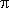we'll receive a central symmetry relatively the origin of coordinates O :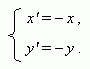A homothetic transformation with a center O ( a , b ) and a coefficient k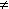0 :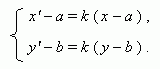An affine transformation: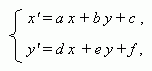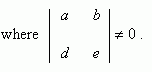An affine transformation transfers straight lines to straight lines, intersecting lines to intersecting lines, parallel straight lines to parallel straight lines. All above mentioned transformations of coordinates are affine transformations.